Скачать презентацию CALCULATIONS FOR NAF BASE MUDS 1 INVERT

CALCULATIONS(Only)-NT.ppt

• Количество слайдов: 17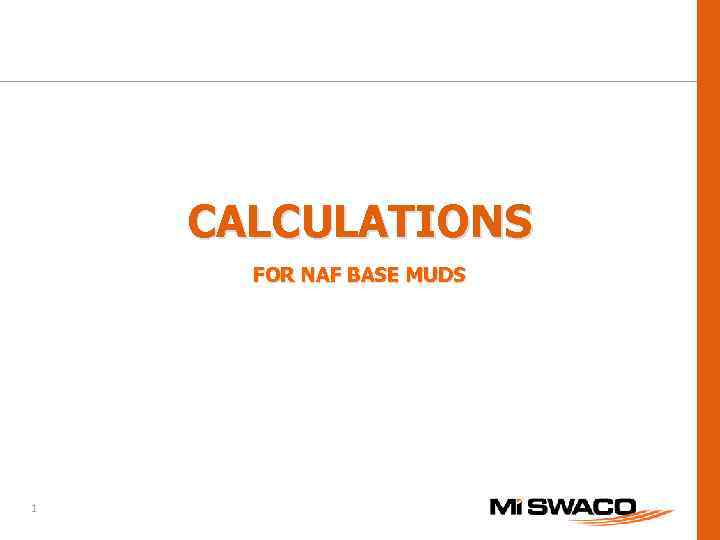CALCULATIONS FOR NAF BASE MUDS 1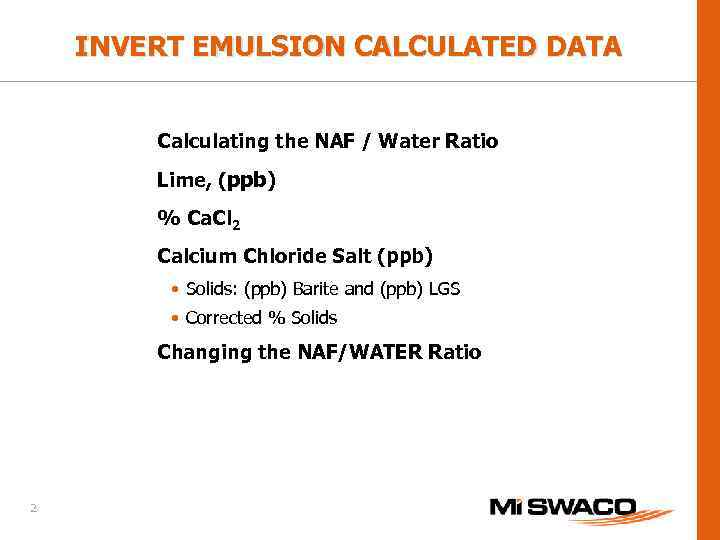INVERT EMULSION CALCULATED DATA Calculating the NAF / Water Ratio Lime, (ppb) % Ca. Cl 2 Calcium Chloride Salt (ppb) • Solids: (ppb) Barite and (ppb) LGS • Corrected % Solids Changing the NAF/WATER Ratio 2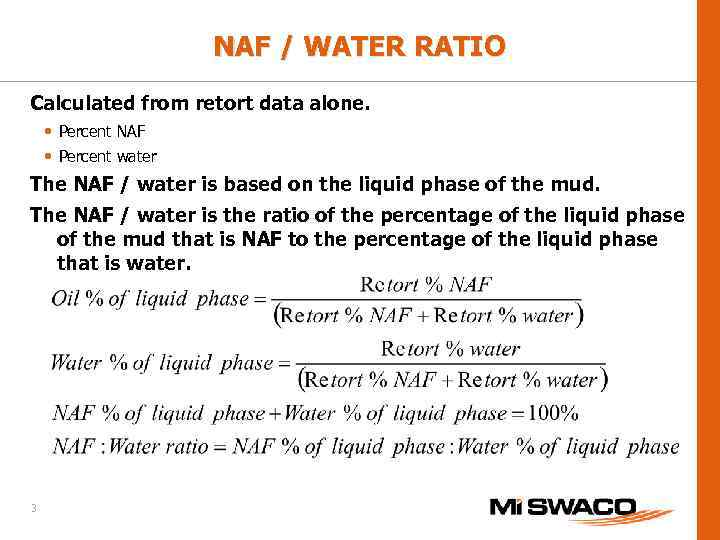NAF / WATER RATIO Calculated from retort data alone. • Percent NAF • Percent water The NAF / water is based on the liquid phase of the mud. The NAF / water is the ratio of the percentage of the liquid phase of the mud that is NAF to the percentage of the liquid phase that is water. 3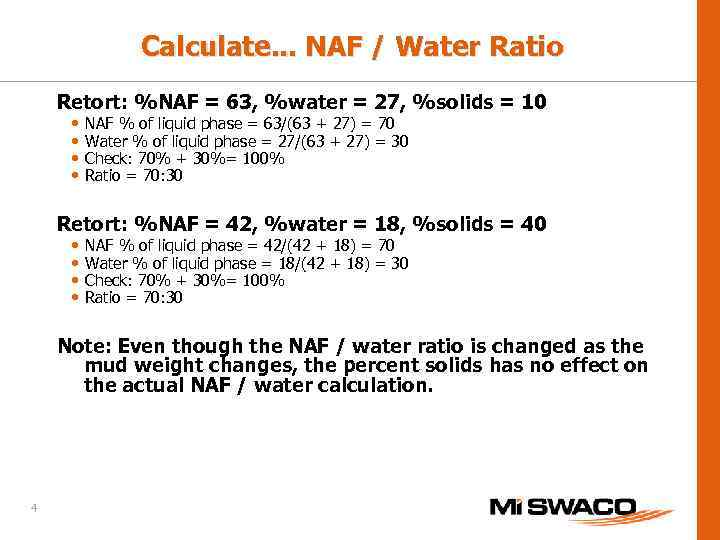Calculate. . . NAF / Water Ratio Retort: %NAF = 63, %water = 27, %solids = 10 • • NAF % of liquid phase = 63/(63 + 27) = 70 Water % of liquid phase = 27/(63 + 27) = 30 Check: 70% + 30%= 100% Ratio = 70: 30 Retort: %NAF = 42, %water = 18, %solids = 40 • • NAF % of liquid phase = 42/(42 + 18) = 70 Water % of liquid phase = 18/(42 + 18) = 30 Check: 70% + 30%= 100% Ratio = 70: 30 Note: Even though the NAF / water ratio is changed as the mud weight changes, the percent solids has no effect on the actual NAF / water calculation. 4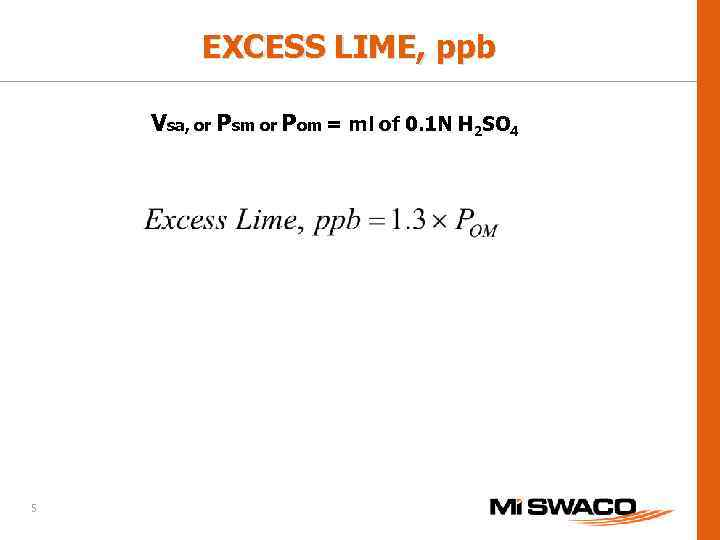EXCESS LIME, ppb Vsa, or Psm or Pom = ml of 0. 1 N H 2 SO 4 5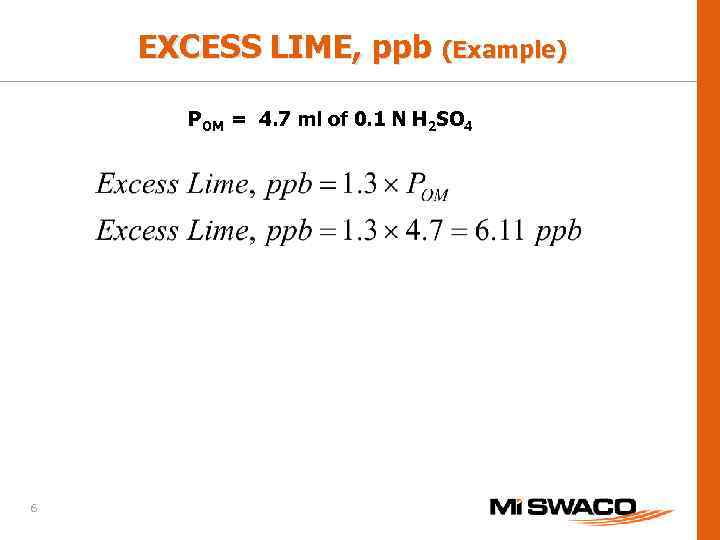EXCESS LIME, ppb (Example) POM = 4. 7 ml of 0. 1 N H 2 SO 4 6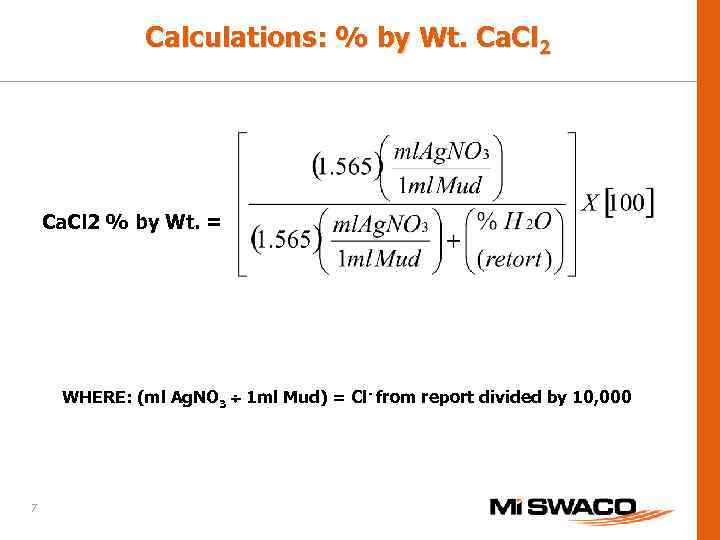Calculations: % by Wt. Ca. Cl 2 % by Wt. = WHERE: (ml Ag. NO 3 1 ml Mud) = Cl- from report divided by 10, 000 7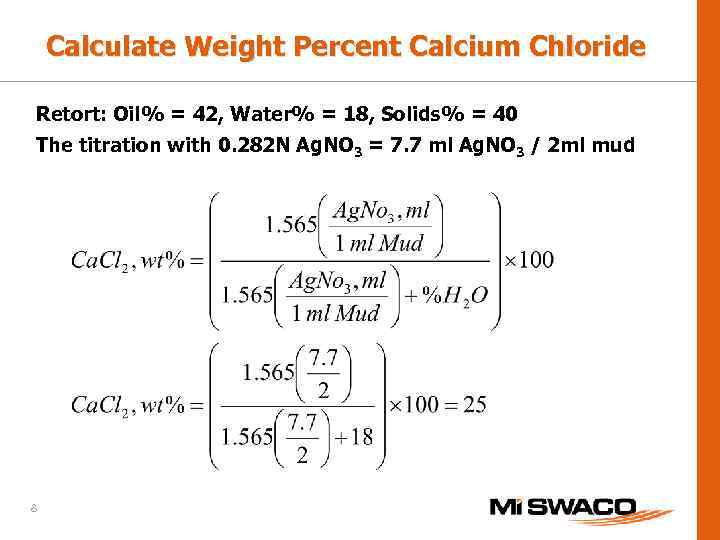Calculate Weight Percent Calcium Chloride Retort: Oil% = 42, Water% = 18, Solids% = 40 The titration with 0. 282 N Ag. NO 3 = 7. 7 ml Ag. NO 3 / 2 ml mud 8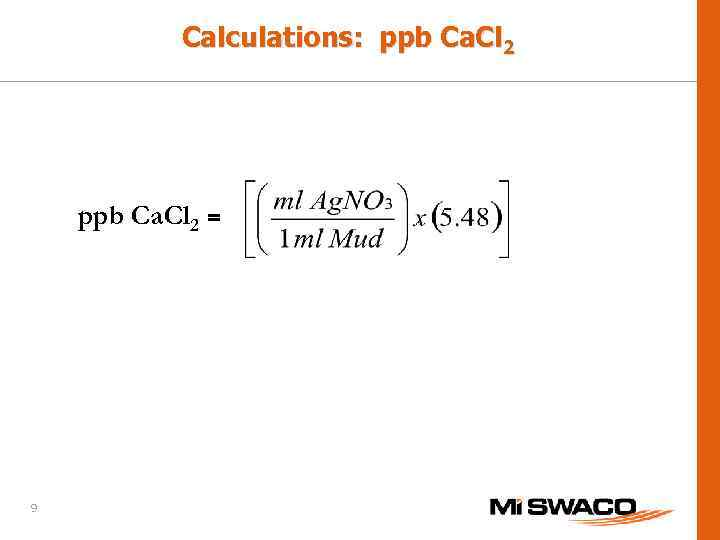Calculations: ppb Ca. Cl 2 = 9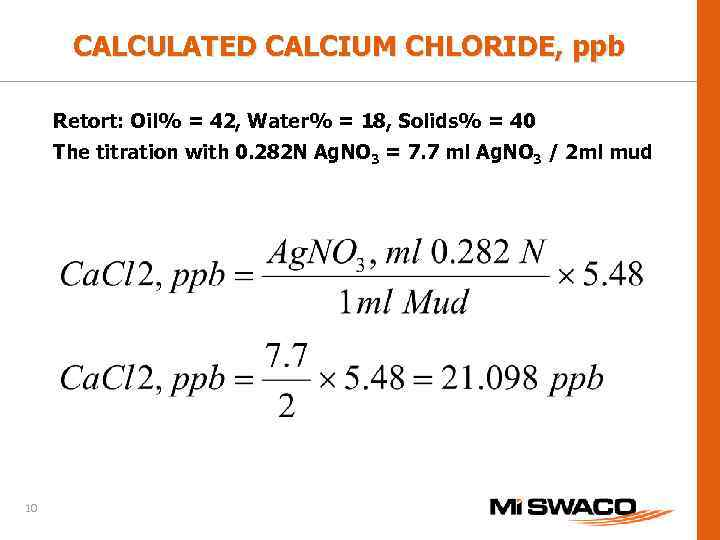CALCULATED CALCIUM CHLORIDE, ppb Retort: Oil% = 42, Water% = 18, Solids% = 40 The titration with 0. 282 N Ag. NO 3 = 7. 7 ml Ag. NO 3 / 2 ml mud 10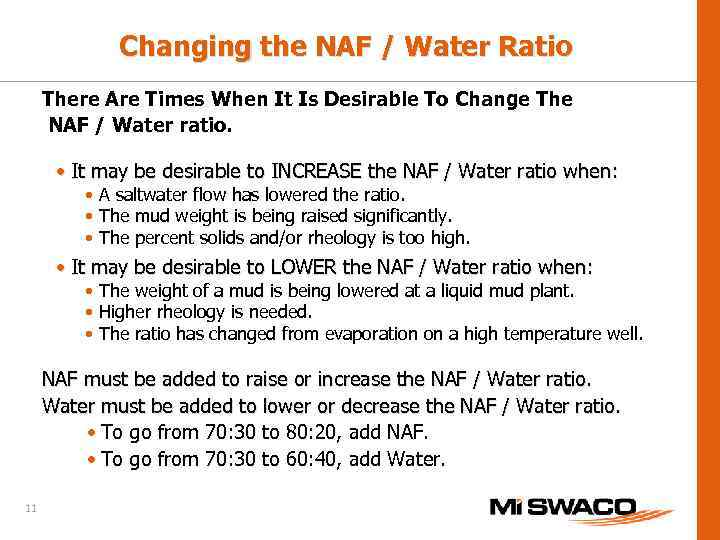Changing the NAF / Water Ratio There Are Times When It Is Desirable To Change The NAF / Water ratio. • It may be desirable to INCREASE the NAF / Water ratio when: • A saltwater flow has lowered the ratio. • The mud weight is being raised significantly. • The percent solids and/or rheology is too high. • It may be desirable to LOWER the NAF / Water ratio when: • The weight of a mud is being lowered at a liquid mud plant. • Higher rheology is needed. • The ratio has changed from evaporation on a high temperature well. NAF must be added to raise or increase the NAF / Water ratio. Water must be added to lower or decrease the NAF / Water ratio. • To go from 70: 30 to 80: 20, add NAF. • To go from 70: 30 to 60: 40, add Water. 11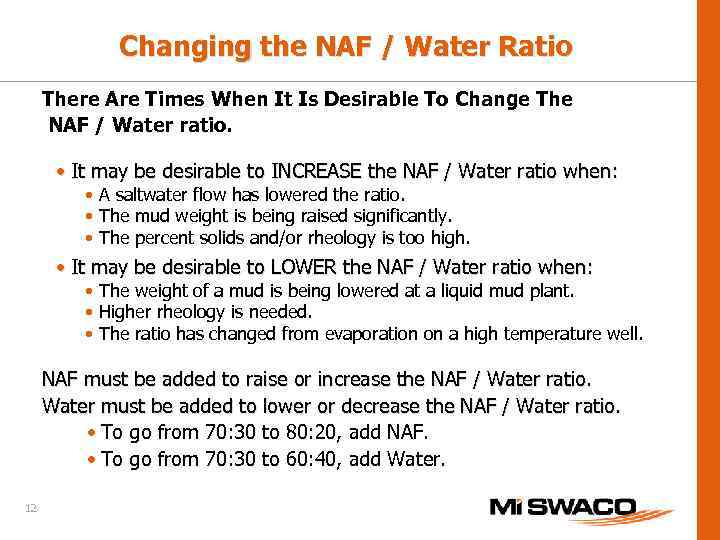Changing the NAF / Water Ratio There Are Times When It Is Desirable To Change The NAF / Water ratio. • It may be desirable to INCREASE the NAF / Water ratio when: • A saltwater flow has lowered the ratio. • The mud weight is being raised significantly. • The percent solids and/or rheology is too high. • It may be desirable to LOWER the NAF / Water ratio when: • The weight of a mud is being lowered at a liquid mud plant. • Higher rheology is needed. • The ratio has changed from evaporation on a high temperature well. NAF must be added to raise or increase the NAF / Water ratio. Water must be added to lower or decrease the NAF / Water ratio. • To go from 70: 30 to 80: 20, add NAF. • To go from 70: 30 to 60: 40, add Water. 12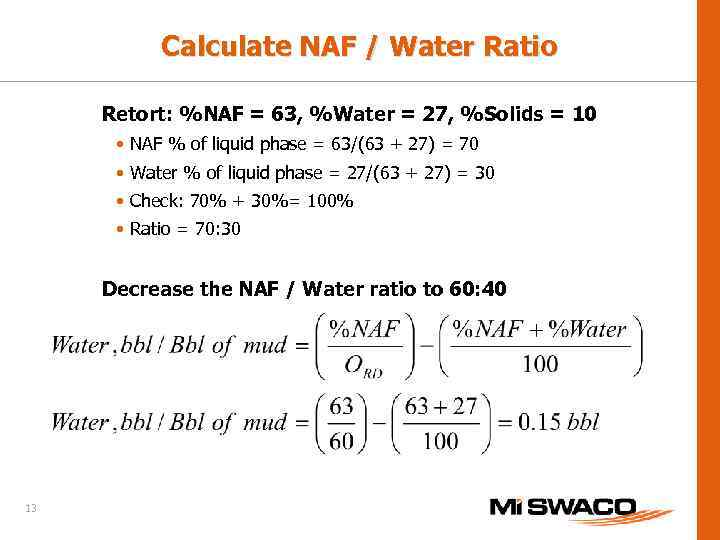Calculate NAF / Water Ratio Retort: %NAF = 63, %Water = 27, %Solids = 10 • NAF % of liquid phase = 63/(63 + 27) = 70 • Water % of liquid phase = 27/(63 + 27) = 30 • Check: 70% + 30%= 100% • Ratio = 70: 30 Decrease the NAF / Water ratio to 60: 40 13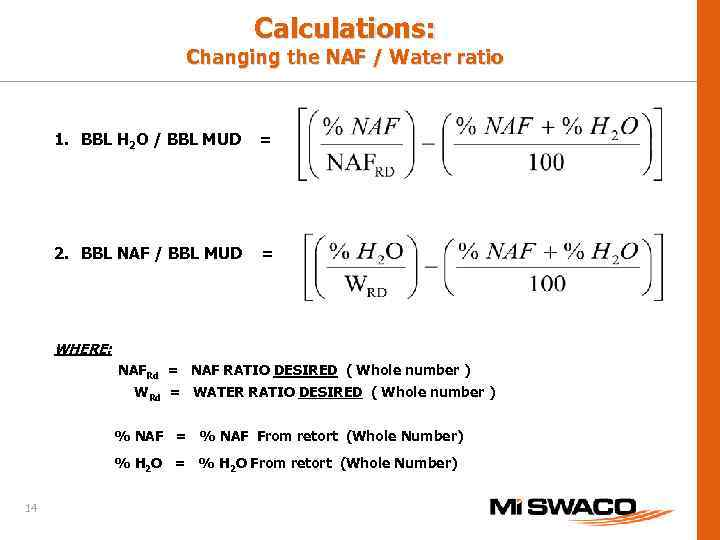Calculations: Changing the NAF / Water ratio 1. BBL H 2 O / BBL MUD = 2. BBL NAF / BBL MUD = WHERE: NAFRd = NAF RATIO DESIRED ( Whole number ) WRd = WATER RATIO DESIRED ( Whole number ) % NAF = % NAF From retort (Whole Number) % H 2 O = % H 2 O From retort (Whole Number) 14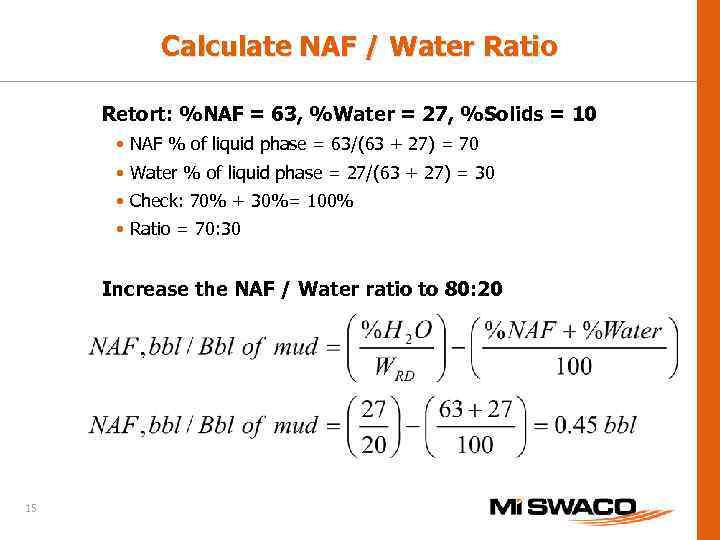Calculate NAF / Water Ratio Retort: %NAF = 63, %Water = 27, %Solids = 10 • NAF % of liquid phase = 63/(63 + 27) = 70 • Water % of liquid phase = 27/(63 + 27) = 30 • Check: 70% + 30%= 100% • Ratio = 70: 30 Increase the NAF / Water ratio to 80: 20 15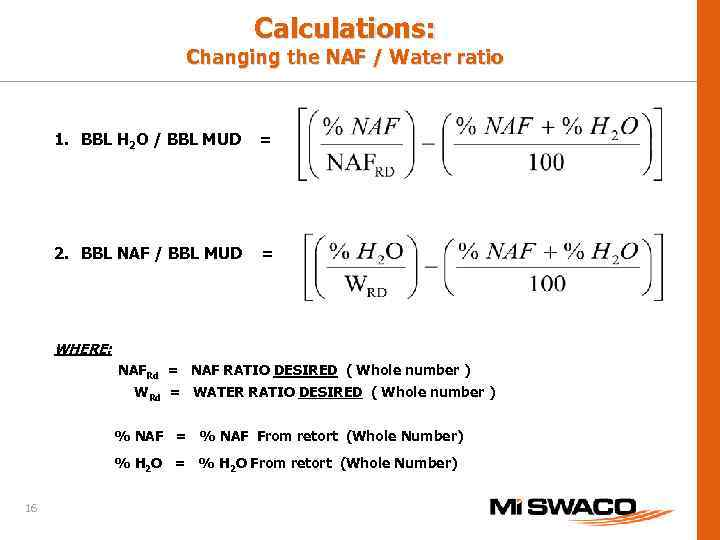Calculations: Changing the NAF / Water ratio 1. BBL H 2 O / BBL MUD = 2. BBL NAF / BBL MUD = WHERE: NAFRd = NAF RATIO DESIRED ( Whole number ) WRd = WATER RATIO DESIRED ( Whole number ) % NAF = % NAF From retort (Whole Number) % H 2 O = % H 2 O From retort (Whole Number) 16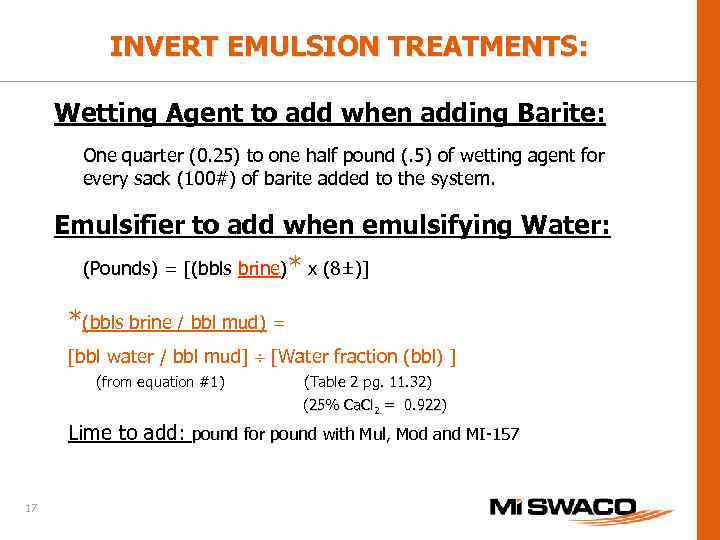INVERT EMULSION TREATMENTS: Wetting Agent to add when adding Barite: One quarter (0. 25) to one half pound (. 5) of wetting agent for every sack (100#) of barite added to the system. Emulsifier to add when emulsifying Water: (Pounds) = [(bbls brine)* x (8±)] *(bbls brine / bbl mud) = [bbl water / bbl mud] [Water fraction (bbl) ] (from equation #1) (Table 2 pg. 11. 32) (25% Ca. Cl 2 = 0. 922) 0. 922 Lime to add: pound for pound with Mul, Mod and MI-157 17